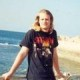# Hindman’s Theorem write-up

It came to my attention that Leo Goldmakher had written up notes for a lecture I gave in August 2011 on the proof of Hindman’s Theorem via ultrafilters. The notes are quite nice so I thought I would share them.

Here is a link to the notes (pdf) and here is Leo’s website.

The lecture I gave follows the papers:

• “An Algebraic Proof of van der Waerden’s Theorem” by Vitaly Bergelson, Hillel Furstenburg, Neil Hindman and Yitzhak Katznelson. (L’enseignement Mathematique, t. 35, 1989, p. 209-215)
• Ultrafilters: Some Old and some New Results” (pdf) by W.W. Comfort. (Bulletin of the AMS, Volume 83, Number 4, July 1977)

## 4 thoughts on “Hindman’s Theorem write-up”

1.saf says:

Cool! Thanks for posting.

Like

2.Ari Brodsky says:

On page 3, the description of the “basis of open sets” for the topology on$U(\mathbb N)$ is not quite right. Describing a collection of open sets requires another set of braces:
$\{ \{\mathcal U \in U(\mathbb N) : A \in \mathcal U \} : A \subseteq \mathbb N \}$

Like

3.Ari Brodsky says:

The proof of Theorem 3.1 relies on several unstated facts that should be explicitly stated (if not proven) in the exposition:

1)$U(\mathbb N)$ is compact.

2)$U(\mathbb N)$ is Hausdorff. [Note that$T_1$ is not enough, because we need all compact subsets to be closed. In fact there is a compact,$T_1$ topological semigroup having no idempotents, namely$(\mathbb N, +)$ with the minimal$T_1$ topology, also known as the finite complement topology.]

3) The operation$\oplus$ is continuous.

Like

1.Ari Brodsky says:

In #3 I meant semicontinuous.

Like